#Quality Digest

Featured Product
This Week in Quality Digest Live
Statistics Features
Alan Metzel
Introducing the Enhanced Perkin Tracker
Donald J. Wheeler
What you think you know may not be so
Tony Boobier
Why data leaders need to master words as well as statistics
Donald J. Wheeler
What they forgot to tell you in your statistics class
Donald J. Wheeler
How does it compare with a process behavior chart?
Statistics News
New capability delivers deeper productivity insights to help manufacturers meet labor challenges
Day and a half workshop to learn, retain, and transfer GD&T knowledge across an organization
Elsmar Cove is a leading forum for quality and standards compliance
InfinityQS’ quality solutions have helped cold food and beverage manufacturers around the world optimize quality and safety
User friendly graphical user interface makes the R-based statistical engine easily accessible to anyone
Collect measurements, visual defect information, simple Go/No-Go situations from any online device
Good quality is adding an average of 11 percent to organizations’ revenue growth
Ability to subscribe with single-user minimum, floating license, and no long-term commitment
A guide for practitioners and managers
Statistics

## An Alternative Test for Randomness of Error Terms in a Regression Model

### There’s more than one way to monitor key variables

Published: Thursday, May 5, 2016 - 11:41Donald S. HolmesA. Erhan Mergen

Regression analysis is used in a variety of manufacturing applications. An example of such an application would be to learn the effect of process variables on output quality variables. This allows the process control people to monitor those key variables and keep the output variables at the desired level.

Regression analysis is also used in design of experiments (DOE) to identify the key process variables that have the most effect on the quality of the end product or service. In addition, if the process is autocorrelated and we want to perform statistical process control (SPC), regression models (i.e., autoregressive models) could help model the autocorrelation in the process and help modify the SPC application accordingly so that the right questions can be tested on the control charts.

One of the issues in regression analysis is checking whether the error terms are random. If the error terms are not random, this could create a problem in interpreting the regression model, since the existence of nonrandomness makes the estimates of the standard errors smaller than the true values (Wilson & Keating1). Durbin-Watson (DW) is a common test, among many, to check if the error terms are random. The Durbin-Watson statistic is compared to a special table value to test the statistical significance of the sample DW result (see, for example, Wilson & Keating1, pp. 182–184 and 234–236).

Another way to test the randomness of the error terms would be a graphical approach, such as using control charts. Once the error terms are generated, the standard deviation of the error terms (s) can be estimated, and control limits can be established around the average of the error terms, which would be zero. Regression models assume that the error terms are independent (i.e., random) and normally distributed with a mean of zero and a constant variance. Thus, to check the randomness of the error terms, the upper and lower control limits (UCL and LCL) can then be calculated as follows:

UCL = 0 + z s and LCL = 0 – z s, where z is the constant picked to determine the distance from the mean in terms of the number of standard deviations. Because a normal distribution is assumed, if we pick z = 3, for example, we will expect that roughly 99.7 percent of the error terms will fall within 0±3s. So, as long as the error terms fall within the established control limits (with no nonrandom patterns), we say the error terms are random. However, error terms could fall within the established limits but display some nonrandom patterns, such as trend and clustering. In this case, the control chart approach can be supplemented by some run tests to detect those nonrandom patterns.

In this article we propose an alternative method to test the randomness of the error terms.

### Proposed method

The proposed method involves comparing two variance estimators: one by using the mean square successive differences (MSSD) and one by using the regular variance formula. This proposed method does not require a special table for a test of significance. We propose using an alternative method that requires only a normal table for tests of significance. The suggested method is based on testing the difference of the two variance estimators of the error terms, i.e., the residuals. The two variance estimators are the regular variance, s2, and the variance estimated using the mean square successive differences (MSSD), q2, which are defined below:(Equation 1)(Equation 2)

where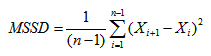(Equation 3)

and n is the number of observations in the data set. Hald2 showed that the estimate obtained through MSSD, i.e., q2, is an unbiased estimate of the usual variance (see Neumann et al.3, 4, Holmes and Mergen5, 6).

These two estimates would be equal (or nearly equal) if the errors are random. To test the significance of the difference between the two estimates, a randomness test given in Dixon and Massey7 can be used, which is: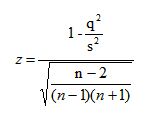(Equation 4)

z has been shown to be approximately normally distributed with a mean of 0 and a variance of 1 (N(0, 1)).

Thus, z values larger than +3 and less than –3 indicate that the two estimates are significantly different, and that the model is not a good fit. On the other hand, z values that fall between +3 and –3 indicate that the difference between the two variance estimates is not significant, i.e., the data set is considered to be random, and the model is a good fit. Hence, if the Xi values above are replaced by error terms (et), i.e., in equations 1 and 3, above, the z statistic defined above may be used to check whether the error terms in regression models are random. To calculate the proposed z statistic as given in equation 4, two variance estimators will be calculated for the error terms, one using the MSSD approach (equations 2 and 3) and the other one using the regular approach (equation 1). z values within ±3 imply that the error terms are random, i.e., do not show any sign of being nonrandom. Because z approximately follows a normal distribution with (0, 1) when n is bigger than 20, the use of z values between ±3 gives approximately a 99.7-percent critical region for the test. The proposed test statistics z given in equation 4 is actually related to a DW statistic. It should be noted that both the definitions of Z and DW involve the ratio of two estimates of variance, but the numerator of the DW needs to be divided by 2 to actually become an estimate of the variance (see equation 5 below).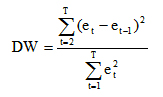(Equation 5)

### Example

The example is from a machine shop that produces brake drums. The data, which are concealed, are on the diameters of sequentially machined hubs. (The data set is included in the appendix below.) The autocorrelation function indicates that autoregressive model with order 1, i.e., AR (1), could be a good fit. The Custom QC software8 that we used indeed picked the AR (1) as the best fit for this data set with the following fitted model: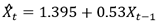(Equation 6)

We then calculated the error terms (i.e., residuals):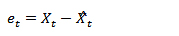(Equation 7)

where Xt is the actual observation andis the estimate at time t, respectively.

To test the significance of this AR (1) model, we used both the DW test and the proposed method on the error terms. The DW statistic and the z value from the proposed method using equations 5 and 4, respectively, are given below:

DW = 2.003 and the z = –0.077.

Both the DW statistic, where the desired value for DW for random variation is 2, and the z value, where the desired value for z is 0, from the proposed method indicate that there is little evidence, if any, of nonrandomness of the errors, i.e., they are random. This also implies that the AR (1) model is a good fit for these data.

The proposed method described here suggests an alternative method for testing whether the error terms in regression models are random. It is not our intent to present this method as a replacement for the DW test, simply as an alternative that does away with the necessity of a special table for testing for significance.

References
1 Wilson, J. H., and Keating, B., Business Forecasting, fifth edition, McGraw-Hill/Irwin, Boston, 2007.
2 Hald, A., Statistical Theory with Engineering Applications, Wiley, New York, 1952.
3 Neumann, J. V., Kent, R. H., Bellinson, H. R., and Hart, B. I., “The Mean Square Successive Difference,” Annals of Mathematical Statistics, 12 (1941), pp. 153–162.
4 Neumann, J. V., “Distribution of the Ratio of the Mean Square Successive Difference to the Variance,” Annals of Mathematical Statistics, 12 (1941), pp. 367–395.
5 Holmes, D. S. and Mergen, A. E., “An Alternative Method to Test for Randomness of a Process,” Quality and Reliability Engineering, International, 11(3), 1995, pp. 171–174.
6 Holmes, D. S. and Mergen, A. E., “An Alternative Method to Test the Residuals in a Regression Model,” The 2008 Northeast Decision Sciences Institute Meeting Proceedings, New York, Mar. 28–30, 2008, pp. 679–684.
7 Dixon, W. J. and Massey, F. J., Introduction to Statistical Analysis, McGraw-Hill, New York, 1969.
8 Custom QC Software, Stochos, Inc., Duanesburg, NY.

Appendix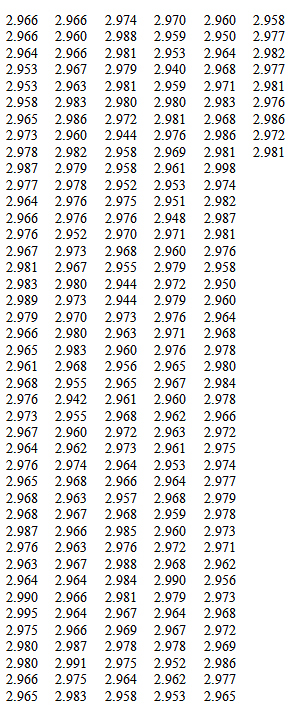### Discuss### Donald S. Holmes

Donald S. Holmes is the president of Stochos Inc., provider of statistical process control consulting and training services. Holmes has retired as a professor from the Graduate Management Institute at Union College in Schenectady, New York. Holmes has bachelor’s and master’s degrees in mathematics. He is a Fellow of the American Society for Quality (ASQ) and an ASQ-certified quality engineer.### A. Erhan Mergen

A. Erhan Mergen is a professor of decision sciences in the Saunders College of Business at the Rochester Institute of Technology, Rochester, New York. Mergen holds a Ph.D. in administrative and engineering systems, a master’s degree in industrial administration, and a bachelor’s degree in management. He is a senior member of the American Society for Quality.

### Type I error

I recognize the Type I error.  I actually cited it in my response, which can't be said for the article.  My question remains.  Why promote +/- 3 for a hypothesis test?  I get it on a single value basis, but not on a hypothesis test of a test statistic.  What you have is a test statistic.  Most of the world chooses 90%, 95% and 99% for confidence levels in hypothesis tests.  Your article doesn't suggest anything about alternatives to +/-3 so the inference is that you believe that +/- 3 is the best choice (or 99.73%).  Why?

### We did not say that we

We did not say that we believe +/- 3 is the best choice (or 99.73%); +/- 3 sigma limits are commonly used in SPC applications (e.g., control charts). When Walter Shewhart introduced the control charts, he stated that +/- 3 sigma limits balances the cost of Type I and Type II errors. Though one can use any limits he/she prefers.

### response

Thanks for the comments. Your comments hold for all the significance tests. The American Statistical Association, for example, recently published a report on the abuse of the p-value (“The ASA's statement on p-values: context, process, and purpose,” by Ronald L. Wasserstein & Nicole A. Lazar, The American Statistician, March 2016). The intent of this paper was not to discuss “the magnitude of a meaningful difference between s² and q²" or develop a power test for the proposed method.  It was merely offering another alternative to the DW test. The issue that you raised and the issue about the power of the test are well taken and could be the topic of another research paper.

### Power of Randomness Tests

Tukey (1991) observed:

Statisticians classically asked the wrong question - and were willing to answer with a lie, one that was often a downright lie. They asked "Are the effects of A and B different?" and they were willing to answer "no".

All we know about the world teaches us that the effects of A and B are always different - in some decimal place - for any A and B. (p. 100)

Most of us know the procedure for guaranteeing that we find a statistically significant difference. We select a huge sample and evaluate the data from this sample. The same effect size (absolute difference between s² and q²) could be statistically significant for a sample of n=50 and not statistically significant for a sample of n=10. The p value provides no information about the magnitude of the effect size (e.g., Cohen, 1994; Cook, 2010).

Thus, "Is the difference between the variance calculated from the sum of squared deviation scores divided by degrees of freedom (s² - the "regular variance" in equation 1) and the variance calculated from the mean square successive differences (q² - the "MSSD variance" in equations 2 & 3) statistically significant?" is not (to my mind) the most important question.

The question that interests me more is "what is the magnitude of a meaningful (concerning) difference between s² and q²". In other words, "What is the magnitude of a 'meaningful' effect size?"

The next question that interests me is "what is the power of the test"? Power refers to the proportion of times we would reject the Null Hypothesis when the effect size has at least a "meaningful" magnitude.

Is there any information concerning the power of tests of the randomness of error terms?

References

Cohen, J. (1994). The Earth is round (p<0.05). American Psychologist, 49(12), 997–1003.

Cook, C. (2010). Five per cent of the time it works 100 per cent of the time: the erroneousness of the P value. Journal of Manual and Manipulative Therapy, 18(3), 123-125.

Tukey, J. W. (1991). The philosophy of multiple comparisons. Statistical Science, 6(1), 100-116.

### Response

Thanks for the comment. You can use +/- 2; we used +/-3 as an example. Just keep in mind that when +/-2 is used, type 1 error would be higher.